# Tag : Angular Material

ItSolutionStuff.com have tutorials for Angular Material tag, here you can study articles of Angular Material tag, Angular Material tag posts collection, most popular and useful tutorials of Angular Material tag, here you can find list of all relevant posts and example about Angular Material tag, we have lists of tutorials and examples about Angular Material tag. very simple and quick example collection of Latest Angular Material tag.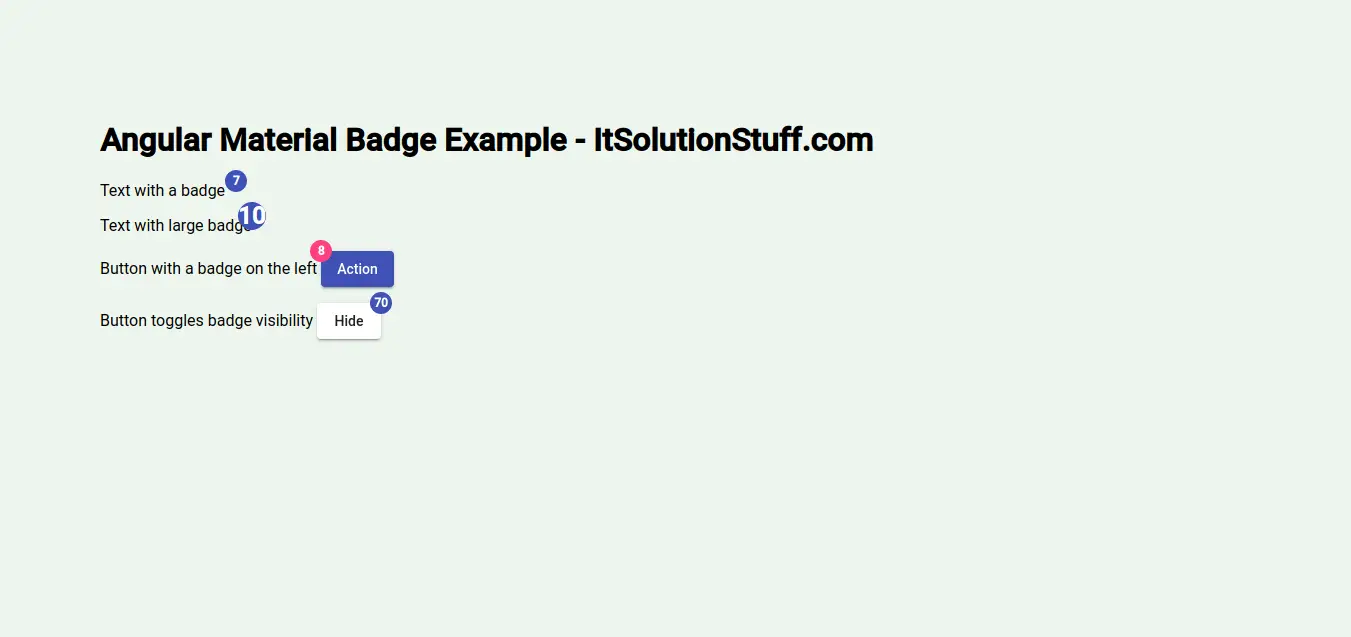## Angular 10 Material Badge Example Tutorial

This article will provide some of the most important example angular 10 material badge example. you will learn angular 10 badge material example. let’s discuss about badge material ui angular 10. step by step explain angular 10 material badge example. you will do the following things for angular 1.....

By Hardik Savani | September 3, 2020 | Category : Angular## Angular 10 Material Card Example

Here, i will show you how to works angular 10 material card example. if you want to see example of angular 10 mat card material then you are a right place. step by step explain angular 10 mat-card example. i would like to show you mat card angular 10 material examples. Angular Material provides a w.....

By Hardik Savani | September 2, 2020 | Category : Angular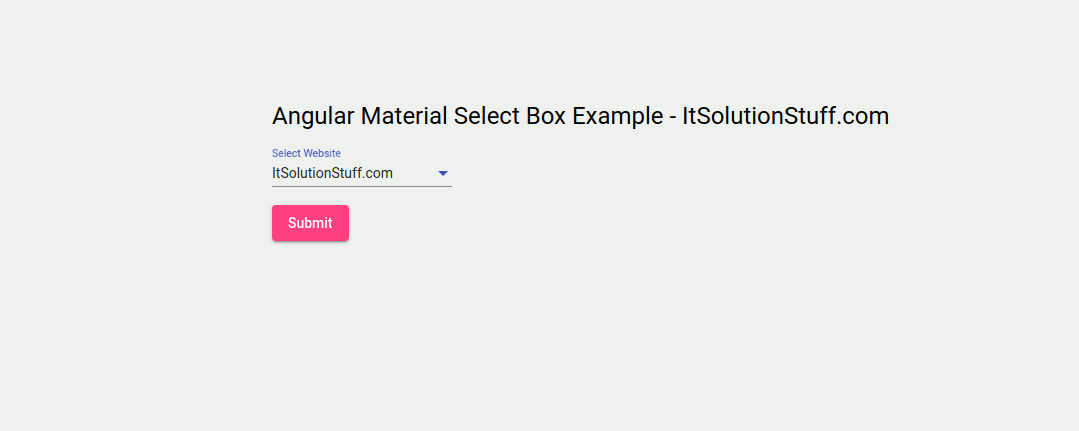## Angular 10 Material Select Dropdown Example

This tutorial is focused on angular 10 material select dropdown example. if you want to see example of angular 10 material select dropdown position then you are a right place. you will learn material select dropdown angular 10. i would like to show you angular 10 material mat select dropdown example.....

By Hardik Savani | August 27, 2020 | Category : Angular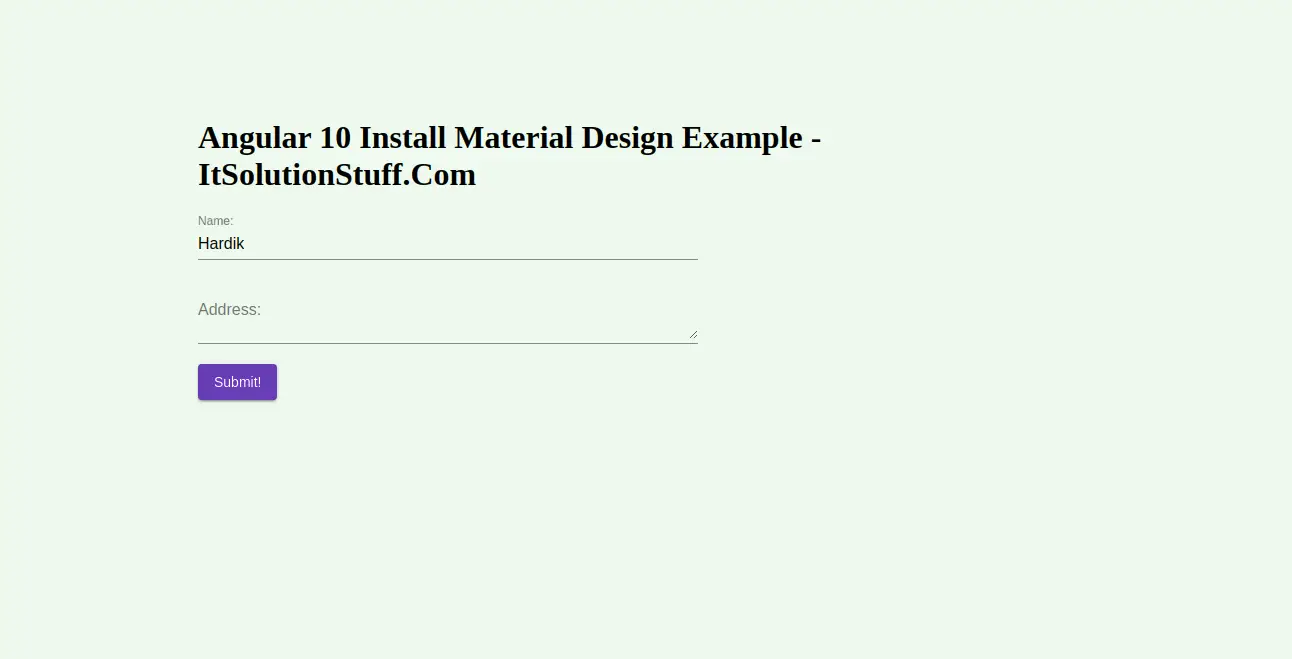## Angular 10 Install Material Design Example

This article will give you example of angular 10 install material design. i would like to share with you how to install angular material in angular 10. This tutorial will give you simple example of how to install material design in angular 10. if you have question about angular 10 install material t.....

By Hardik Savani | August 13, 2020 | Category : Angular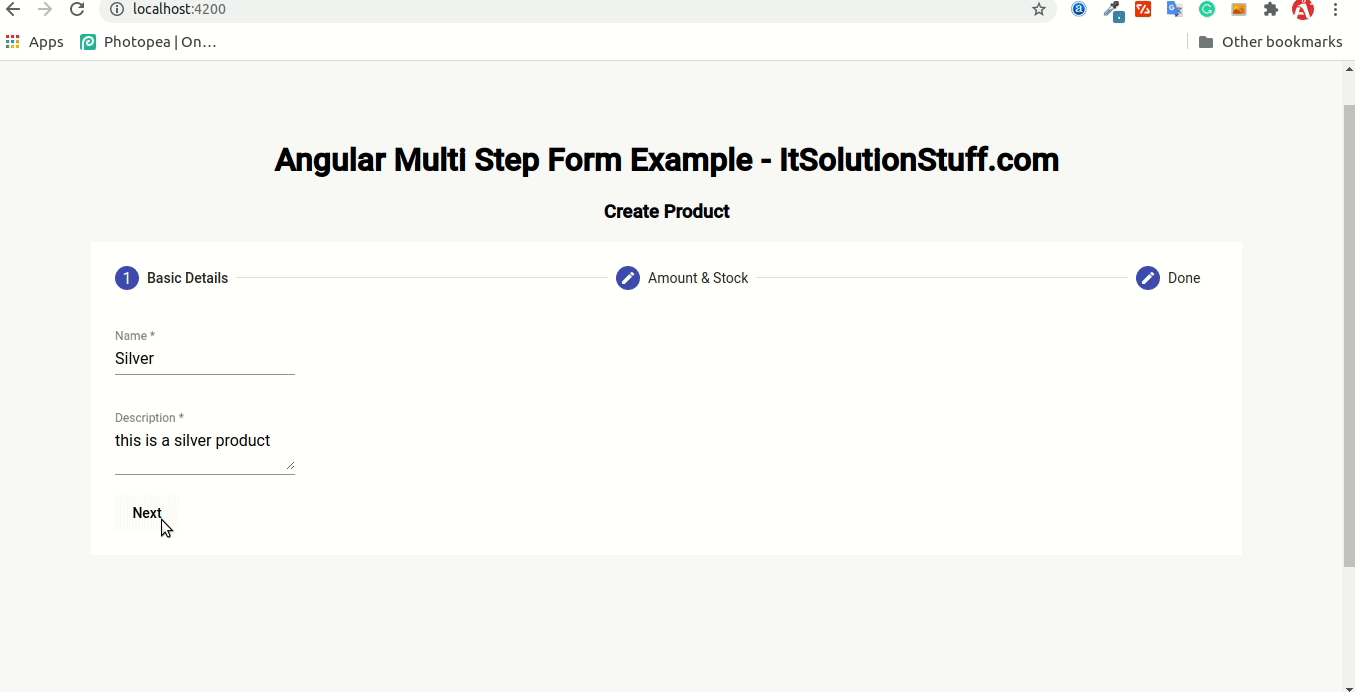## Angular Material Multi Step Form Example

In this tutorial, we will discuss angular material multi step form. if you have question about angular material multi step reactive form then i will give simple example with solution. this example will help you how to create multi step form in angular material. you will learn multi step form angular.....

By Hardik Savani | August 3, 2020 | Category : Angular## Angular Material Selection List Example | Angular 9 mat-selection-list

Here, i will show you angular material selection list example. you can understand a concept of angular material mat-selection-list example. it's simple example of angular material list example. you can see mat-selection-list angular material. Angular Material List component will provide a wraps and.....

By Hardik Savani | July 29, 2020 | Category : Angular## Angular Material Expansion Panel Example | Angular 9 mat-expansion-panel

Now, let's see example of angular material expansion panel example. this example will help you angular 9 material expansion panel. This post will give you simple example of angular mat-expansion-panel example. This article will give you simple example of angular mat expansion panel example. Angular.....

By Hardik Savani | July 28, 2020 | Category : Angular## Angular Material Divider Example | Angular 9 mat-divider

In this example, i will show you angular material divider example. you can understand a concept of angular material vertical divider example. you will learn angular 9 material divider example. this example will help you divider angular material. Angular Material Divider component will allow you to .....

By Hardik Savani | July 23, 2020 | Category : Angular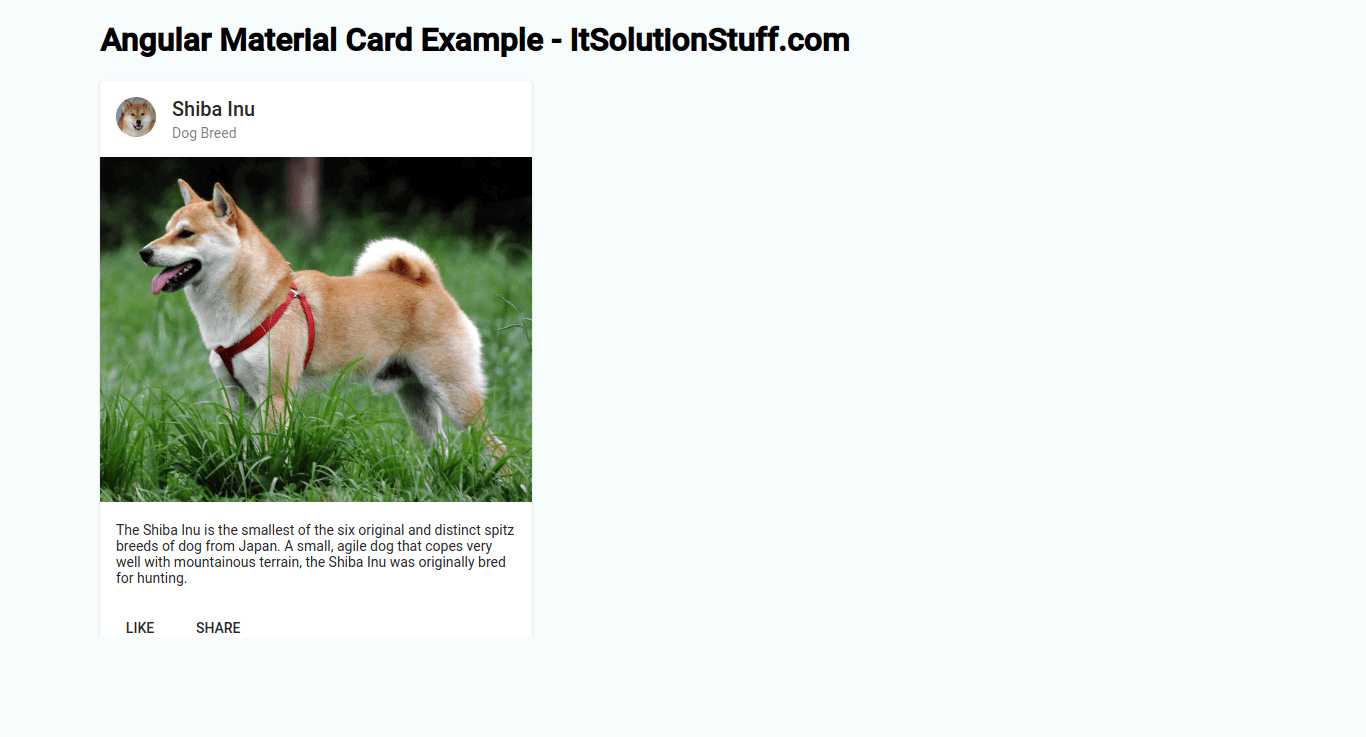## Angular Material Card Example | Angular 9 mat-card

Hi Dev, In this example, i will show you angular material card example. this example will help you angular 9 material card example. Here you will learn angular material mat-card example. you'll learn angular material card grid example. Angular Material provides a wide range of web components which .....

By Hardik Savani | July 22, 2020 | Category : Angular